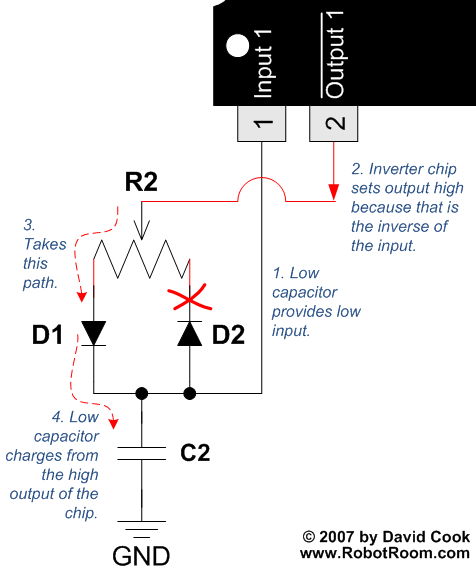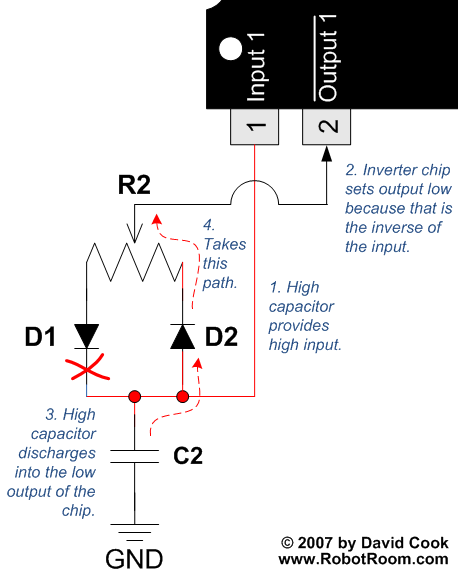• Home• Patreon• Links• Books
Pulse-Width Modulation:

# 2. Charging and Discharging a Capacitor to Create a PWM Wave

(article continued from previous page)

To understand how the 74AC14 PWM circuit works, let’s zoom in on the section of the schematic containing the trimpot, diodes, capacitor, and the first inverter logic gate.

## Capacitor Charge CyclePartial schematic showing the charging path of a variable duty-cycle PWM circuit.

1. The capacitor is empty (discharged) when the power is first applied to the circuit. Because the capacitor is low, and because the capacitor is connected to the input pin of the inverter gate, the input is low.
2. An inverter gate produces an output that is opposite of the input. Because the input is currently low, the output is high.
3. The conventional current flows from the output of the inverter gate through the left half of the potentiometer (R2) and then through diode D1. The current cannot flow through the right half of the variable resistor (R2) because diode D2 blocks the path (notice the direction of the diode arrow). Diodes are one-way valves.
4. The current flows into the capacitor (C2); charging the capacitor. If the potentiometer is dialed to the far left, the resistance will be small and the capacitor will charge quickly. If the potentiometer is dialed to the far right, the resistance will be large and the capacitor will charge slowly.

## Capacitor Discharge CyclePartial schematic showing the charging path of a variable duty-cycle PWM circuit.

1. Eventually, the capacitor fills up (is charged). Because the capacitor is now high, and because the capacitor is connected to the input pin of the inverter gate, the input is now high.
2. An inverter gate produces an output that is opposite of the input. Because the input is now high, the output is switched to low.
3. The high capacitor (C2) now begins to discharge into the low output pin of the inverter.
4. The conventional current flows from the capacitor (C2), through diode D2, and then through the right half of the potentiometer (R2). If the potentiometer is dialed to the far right, the resistance will be small and the capacitor will discharge quickly. If the potentiometer is dialed to the far left, the resistance will be large and the capacitor will charge slowly.

## How Pulse-Width Modulation Works

The repeated charging and discharging of the capacitor occurs because the inverter gate output flips to the opposite of the state of the capacitor. When the capacitor is empty, the inverter output charges the capacitor. When the capacitor is full, the inverter output discharges the capacitor.

If this were the only feature of this circuit, the output would be “on” half of the time (when charging the capacitor) and “off” half of the time. This would provide 50% power (only “on” half the time) to whatever was connected.

The trick is that the resistance of the “on” path is different from the “off” path due to the diodes. That means you can dial the trimpot to make the “on time” take more or less time than the “off time”. As such, you can supply an output signal that is “on” from almost 0% to 100% of the time.

Obviously if the outputs are “on” only 10% of the time, the attached device is only going to be “on” only 10% of the time. In this way, the total amount of power delivered to an attached device can be varied by simply turning the potentiometer to alter the on/off times (duty cycle).

It may help to see the actual outputs on an oscilloscope...